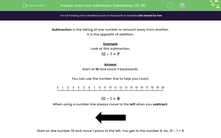# Know Your Subtraction: Subtract 1, 2 or 3 From a Number

In this worksheet, students will practise subtracting the numbers 1, 2 or 3 from another number. (0-20)Key stage:  KS 1

Curriculum topic:   Number: Addition and Subtraction

Curriculum subtopic:   Add and Subtract to 20

Difficulty level:#### Worksheet Overview

Subtraction is the taking of one number or amount away from another.

It is the opposite of addition.

Example

Look at this subtraction.

10 - 1 = ?

Start at 10 and count 1 backwards.10 - 1 = 9

When using a number line always move to the left when you subtract.Start on the number 10 and move 1 place to the left. You get to the number 9. So, 10 - 1 = 9

What if you wanted to subtract 2 from a number?

Example

12 - 2 = ?

Start at 12 and count 2 backwards. So, start at the number 12 on the number line and count back 2 places to the left.

You get to the number 10.

12 - 2 = 10

Are you ready to practise some now and become a maths wizard?### What is EdPlace?

We're your National Curriculum aligned online education content provider helping each child succeed in English, maths and science from year 1 to GCSE. With an EdPlace account you’ll be able to track and measure progress, helping each child achieve their best. We build confidence and attainment by personalising each child’s learning at a level that suits them.

Get started